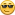## How to expand the control leds on PIC-MAXI-WED?

Started by phthtung, March 15, 2016, 04:49:15 AM

#### phthtung

I want to add the LED driver but when control led10 it came back led1 (not control led10)?, despite repair code in the file:
HWP...h:
// I/O pins
#define LED0_TRIS         (TRISJbits.TRISJ0)
#define LED0_IO            (PORTJbits.RJ0)
#define LED1_TRIS         (TRISHbits.TRISH3)
#define LED1_IO            (PORTHbits.RH3)
#define LED2_TRIS         (TRISBbits.TRISB0)
#define LED2_IO            (PORTBbits.RB0)
#define   LED3_TRIS         (TRISBbits.TRISB1)
#define LED3_IO            (PORTBbits.RB1)
#define   LED4_TRIS         (TRISBbits.TRISB2)
#define   LED4_IO            (PORTBbits.RB2)
#define   LED5_TRIS         (TRISBbits.TRISB3)
#define   LED5_IO            (PORTBbits.RB3)
#define   LED6_TRIS         (TRISGbits.TRISG6)
#define   LED6_IO            (PORTGbits.RG6)
#define   LED7_TRIS         (TRISGbits.TRISG5)
#define   LED7_IO            (PORTGbits.RG5)
#define   LED8_TRIS         (TRISGbits.TRISG4)
#define   LED8_IO            (PORTGbits.RG4)
#define   LED9_TRIS         (TRISFbits.TRISF7)
#define   LED9_IO            (PORTFbits.RF7)
#define   LED10_TRIS         (TRISGbits.TRISG7)
#define   LED10_IO         (PORTGbits.RG7)

CustomHTTPApp.c:
ptr = HTTPGetROMArg(curHTTP.data, (ROM BYTE *)"led10");
if(ptr)
LED10_IO = (*ptr == '1');
ptr = HTTPGetROMArg(curHTTP.data, (ROM BYTE *)"led9");
if(ptr)
LED9_IO = (*ptr == '1');
ptr = HTTPGetROMArg(curHTTP.data, (ROM BYTE *)"led8");
if(ptr)
LED8_IO = (*ptr == '1');
ptr = HTTPGetROMArg(curHTTP.data, (ROM BYTE *)"led7");
if(ptr)
LED7_IO = (*ptr == '1');
ptr = HTTPGetROMArg(curHTTP.data, (ROM BYTE *)"led6");
if(ptr)
LED6_IO = (*ptr == '1');
ptr = HTTPGetROMArg(curHTTP.data, (ROM BYTE *)"led5");
if(ptr)
LED5_IO = (*ptr == '1');
ptr = HTTPGetROMArg(curHTTP.data, (ROM BYTE *)"led4");
if(ptr)
LED4_IO = (*ptr == '1');
ptr = HTTPGetROMArg(curHTTP.data, (ROM BYTE *)"led3");
if(ptr)
LED3_IO = (*ptr == '1');
ptr = HTTPGetROMArg(curHTTP.data, (ROM BYTE *)"led2");
if(ptr)
LED2_IO = (*ptr == '1');
ptr = HTTPGetROMArg(curHTTP.data, (ROM BYTE *)"led1");
if(ptr)
LED1_IO = (*ptr == '1');
.......................
MainDemo.C:
// LEDs
LED0_TRIS = 0;
LED1_TRIS = 0;
LED2_TRIS = 0;
LED3_TRIS = 0;
LED4_TRIS = 0;
LED5_TRIS = 0;
LED6_TRIS = 0;
LED7_TRIS = 0;
LED8_TRIS = 0;
LED9_TRIS = 0;
LED10_TRIS = 0;
Index.htm:
<a id="led1" onclick="newAJAXCommand('leds.cgi?led=1');">&bull;</a>
<a id="led2" onclick="newAJAXCommand('leds.cgi?led=2');">&bull;</a>
<a id="led3" onclick="newAJAXCommand('leds.cgi?led=3');">&bull;</a>
<a id="led4" onclick="newAJAXCommand('leds.cgi?led=4');">&bull;</a>
<a id="led5" onclick="newAJAXCommand('leds.cgi?led=5');">&bull;</a>
<a id="led6" onclick="newAJAXCommand('leds.cgi?led=6');">&bull;</a>
<a id="led7" onclick="newAJAXCommand('leds.cgi?led=7');">&bull;</a>
<a id="led8" onclick="newAJAXCommand('leds.cgi?led=8');">&bull;</a>
<a id="led9" onclick="newAJAXCommand('leds.cgi?led=9');">&bull;</a>
<a id="led10" onclick="newAJAXCommand('leds.cgi?led=10');">&bull;</a>
Status.xlm:
<led0>~led(0)~</led0>
<led1>~led(1)~</led1>
<led2>~led(2)~</led2>
<led3>~led(3)~</led3>
<led4>~led(4)~</led4>
<led5>~led(5)~</led5>
<led6>~led(6)~</led6>
<led7>~led(7)~</led7>
<led8>~led(~</led8>
<led9>~led(9)~</led9>
<led10>~led(10)~</led10>

Looking forward to your help. Thanks.

#### kyrk.5

#1
Hi,

I think here are some lines missing from your post:
CustomHTTPApp.c:
... <= here
LED10_IO = (*ptr == '1');
ptr = HTTPGetROMArg(curHTTP.data, (ROM BYTE *)"led9");
if(ptr)

Could you please add this missing lines to the post, so that we can be sure that the check is properly implemented?

But I think the check will be fine, and the problem is hidden somewhere. I think on the following thing: Everything works fine until the LED numbers are 1 digits. When a LED with two digits came into play, then some buffer is not properly erased or handled and that is why somewhere in the software a check is thinking that it found LED 10 but in reality it is LED1 (plus a remaining 0 from the last check). So you should debug the function HTTPGetROMArg and look for a buffer which is not complety erased.

As an alternative (workaround) you can try to renam the LED from led10 to leda, to see if it is working. I guess it will.

#2
Thanks